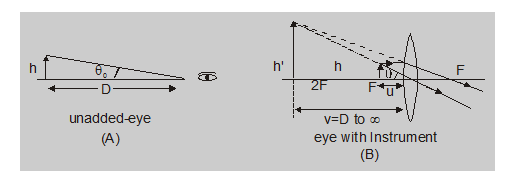Most Affordable JEE | NEET | 8,9,10 Preparation by Kota's Top IITian Doctor Faculties

# What is simple microscope - Construction, Working - eSaralHey, do you want to know what is simple microscope? If yes. Then keep reading

## Microscope

It is an optical instrument used to increase the visual angle of neat objects which are too small to be seen by the naked eye.

## Simple Microscope

It is also known as a magnifying glass or simply magnifier and consists of a convergent lens with the object between its focus and optical center and eye close to it. The image formed by it is erect, virtually enlarged and on the same side of the lens between object and infinity.The magnifying power (MP) or angular magnification of a simple microscope (or an optical instrument) is defined as the ratio of visual angle with an instrument to the maximum visual angle for clear vision when the eye is unadded (i.e., when the object is at least distance of distinct vision)

i.e.,

$\mathrm{MP}=\frac{\text { Visual angle with instrument }}{\text { Max. visual angle for unadded eye }}$

$=\frac{\theta}{\theta_{0}}$

If an object of size h is placed at a distance u ( D) from the eye

$\theta=\frac{h^{\prime}}{v}=\frac{h}{u}$

with $\theta_{0}=\frac{\mathrm{h}}{\mathrm{D}}$

So $\quad \mathrm{MP}=\frac{\theta}{\theta_{0}}=\frac{\mathrm{h}}{\mathrm{u}} \times \frac{\mathrm{D}}{\mathrm{h}}=\frac{\mathrm{D}}{\mathrm{u}}$....(1)

Now there are two possibilities ­

$\left(a_{1}\right)$ If their image is at infinity [Far point]

In this situation from lens formula –

$\frac{1}{v}-\frac{1}{u}=\frac{1}{f}$

we have $\frac{1}{\infty}-\frac{1}{-\mathrm{u}}=\frac{1}{\mathrm{f}}$

i.e., $\mathrm{u}=\mathrm{f}$

So $M P=\frac{D}{U}=\frac{D}{f}$......(2)

As here u is maximum [as object is to be with in focus], MP is minimum and as in this situation parallel beam of light enters the eye, eye is least strained and is said to be normal, relaxed or unstrained.

$\left(a_{2}\right)$ If the image is at D [Near point]

In this situation as v = D, from lens formula

$\frac{1}{v}-\frac{1}{u}=\frac{1}{f}$

we have $\frac{1}{-\mathrm{D}}-\frac{1}{-\mathrm{u}}=\frac{1}{\mathrm{f}}$

i.e., $\frac{D}{u}=1+\frac{D}{f}$

So $\mathrm{MP}=\frac{\mathrm{D}}{\mathrm{u}}=\left[1+\frac{\mathrm{D}}{\mathrm{f}}\right]$....(3)

As the minimum value of v for clear vision is D, in this situation u is minimum and hence this is the maximum possible MP of a simple microscope and as in this situation final image is closest to the eye, the eye is under maximum strain.

Special POints :

1. A simple magnifier is an essential part of most optical instruments (such as a microscope or telescope) in the form of eyepiece or ocular.

2. The magnifying power (MP) has no unit. It is different from the power of a lens which is expressed in diopter (D) and is equal to the reciprocal of focal length in meter.

3. With the increase in wavelength of light used, the focal length of the magnifier will increase, and hence its MP will decrease.

So, that's all from this article. I hope you get the idea about what is a simple microscope. If you found this article informative then please share it with your friends. If you have any confusion related to this topic then you can ask in the comments section down below.

For a better understanding of this chapter, please check the detailed notes of Ray Optics. To watch Free Learning Videos on physics by Saransh Gupta sir Install the eSaral App.